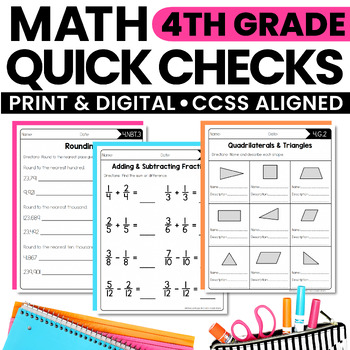digital

# Math Quick Checks - 4th Grade | Digital Pages Google Slides | Distance LearningSubject
Resource Type
Format
PDF (4 MB|79 pages)Google Apps™
Standards
\$5.50
\$5.50The Teacher-Author indicated this resource includes assets from Google Workspace (eg. docs, slides, etc.).

#### Also included in

1. What are Interactive Math Notebooks? Interactive math notebooks are teaching pages used to introduce and teach new math concepts and skills. Each set of notebook pages includes definitions, explanations, rules, and/or algorithms that may be needed to address each standard. Sample and practice proble
\$25.50
\$31.50
Save \$6.00
2. This BUNDLE includes everything you need for 4th grade math!!★ Interactive Notebook Pages★ Math Quick Checks ★ Math Menus (Perfect for homework or early finishers!!)★ Open-Ended Math Pages★ Math Mastery Task Cards★ Units for Fractions, Decimals, Measurement & GeometryFeel free to email with any
\$82.50
\$102.70
Save \$20.20

### Description

These Math Quick Checks are Common Core aligned. They are exactly what you need to quickly check for understanding or assess student learning. They can be used as assessments, exit tickets, homework, or to help reteach in small groups.

These Quick Checks were originally created to pair with my Interactive Math Notebooks, but alone they are still a great tool to use when teaching Common Core Math Standards.

Includes Digital Pages to use with Google Slides.

Here is a list of titles for each of the worksheets, along with the standard:

Multiplication Equations as Comparisons (4.OA.1)

Word Problems with Multiplicative Comparison (4.OA.2)

Multi-Step Word Problems (4.OA.3)

Multiples & Factors (4.OA.4)

Prime & Composite Number (4.OA.4)

Patterns (4.OA.5)

Place Value (4.NBT.1)

Numerals, Word Form, and Expanded Form (4.NBT.2)

Comparing Numbers (4.NBT.2)

Rounding Numbers (4.NBT.3)

Multiplication – By 1 Digit (4.NBT.5)

Multiplication – By 2 Digits (4.NBT.5)

Division (4.NBT.6)

Equivalent Fractions (4.NF.1)

Comparing Fractions (4.NF.2)

Decomposing Fractions (4.NF.3)

Adding & Subtracting Mixed Numbers (4.NF.3)

Word Problems: Adding & Subtracting Fractions (4.NF.3)

Multiplying Fractions by Whole Numbers (4.NF.4)

Word Problems: Multiplying Fractions by Whole Numbers (4.NF.4)

Fractions with Denominators of 10 & 100 (4.NF.5)

Relating Fractions & Decimals (4.NF.6)

Comparing Decimals (4.NF.7)

Relative Sizes of Measurement (4.MD.1)

Measurement Conversions (4.MD.1)

Word Problems: Measurement (4.MD.2)

Word Problems: Area & Perimeter (4.MD.3)

Line Plots (4.MD.4)

Understanding Angles (4.MD.5)

Measuring & Sketching Angles (4.MD.6)

Unknown Angles (4.MD.7)

Points, Lines, Line Segments & Rays (4.G.1)

Angles (4.G.1)

Perpendicular & Parallel Lines (4.G.1)

Lines of Symmetry (4.G.3)

There is an answer key for each page.

Feel free to email me at rebecca@createteachshare.com with any questions or comments.

This product is also available in a BUNDLE!!!

4th Grade Interactive Math Notebook {BUNDLE}

★★This resources is included in my ULTIMATE 4th Grade Math Bundle!!★★

Check out all of my 4th Grade Interactive Math Notebooks, Worksheets, & Activities:

4th Grade Interactive Math Notebook: OA

4th Grade Interactive Math Notebook: NBT

4th Grade Interactive Math Notebook: NF

4th Grade Interactive Math Notebook: MD

4th Grade Interactive Math Notebook: G

Common Core Math Worksheets (for all 4th grade standards)

Solve 'n' Sequence Math Activities

Total Pages
79 pages
Included
Teaching Duration
1 Year
Report this Resource to TpT
Reported resources will be reviewed by our team. Report this resource to let us know if this resource violates TpT’s content guidelines.

### Standards

to see state-specific standards (only available in the US).
Compare two decimals to hundredths by reasoning about their size. Recognize that comparisons are valid only when the two decimals refer to the same whole. Record the results of comparisons with the symbols >, =, or <, and justify the conclusions, e.g., by using a visual model.
Use decimal notation for fractions with denominators 10 or 100. For example, rewrite 0.62 as 62/100; describe a length as 0.62 meters; locate 0.62 on a number line diagram.
Express a fraction with denominator 10 as an equivalent fraction with denominator 100, and use this technique to add two fractions with respective denominators 10 and 100. For example, express 3/10 as 30/100, and add 3/10 + 4/100 = 34/100.
Recognize a line of symmetry for a two-dimensional figure as a line across the figure such that the figure can be folded along the line into matching parts. Identify line-symmetric figures and draw lines of symmetry.
Classify two-dimensional figures based on the presence or absence of parallel or perpendicular lines, or the presence or absence of angles of a specified size. Recognize right triangles as a category, and identify right triangles.